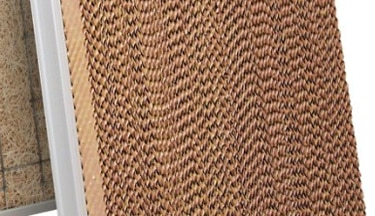# Efficiency Numbers: What they mean and how they relate to our various products

Author: Gary Jouas –
Evaporative Cooling Efficiency is the ratio of the actual cooling produced, to the energy required supplying it, relative to the theoretical maximum that could be produced, expressed in percentage. When discussing the efficiency of evaporative cooling media we are really focused on the actual temperature drop as the air passes through the media. In order to begin understanding this concept, you must first understand a few relevant terms:

1. Dry Bulb Temperature (Db): This is the ambient air temperature that surrounds you.
2. Wet Bulb Temperature (Dw): This is lowest temperature the air can attain by evaporating water into the air.
3. Wet Bulb Depression (∆T): This is the difference between the Dry Bulb and Wet Bulb temperature. (Db – Dw=∆T)
4. Efficiency (eff): This is a ratio of the actual air temperature drop across the media compared to the Wet Bulb Depression, expressed as a decimal percentage. If the Wet Bulb Depression is 40 degrees and the actual temperature drop measured across the cooling media is 30 degrees, the cooling efficiency of the media is 75%  (30/40 = .75).  This cooling efficiency is also known as the “Saturation Efficiency” because it refers to the amount of moisture that the media can evaporate into the air.

Now that we understand the terms, let’s discuss how this relates to evaporative media and how that might affect your decision on which media will work best for your application.

To determine the outlet air temperature of a cooler, you must multiply the “Wet Bulb Depression” x “efficiency”, then subtract that from the “Dry Bulb Temperature”.

• Outlet Air = Db – (∆T x eff)

That expression illustrates the importance of a higher efficiency number in that the closer the efficiency gets to 100% (or 1), the larger the number that is to be subtracted from the Dry Bulb Temperature; hence a greater the cooling effect.

To further illustrate the effects of greater efficiency, note the comparison below using typical saturation efficiencies of different media. For illustration purposes we will choose the following conditions:

• Db=100°F
• Wb=60°F
• ∆T=40°F
1. Aspen Media @ 75% efficient, the outlet air temperature will be 70°F. 100°F
-(40°F x .75)=70°F
2. 8”Rigid Media @ 85% efficient, the outlet air temperature will be 66°F.
(100°F-(40°F x .85)=66°F
3. 12”Rigid Media @ 89% efficient, the outlet air temperature will be 64.4°F.
(100°F-(40°F x .89)=64.4°F
4. 4×4 Rigid Media @ 93% efficient, the outlet air temperature will be 62.8°F.
(100°F-(40°F x .93)=62.8°FAs you can see by comparing the outlet air temperatures, the 4×4 media, is the right choice for maximum cooling effect. Outlet air temperature is by far the primary consideration; however there are other variables that can affect your particular application. The following are a few quick references:

• Aspen Media:
• 75% efficient
• Least restrictive to air flow
• Media should be replaced each year
• 8” Rigid Media:
• 85% efficient
• More restrictive to air flow than Aspen Media
• Media can last 5 to 10-years prior to required replacement
• 12” Rigid Media:
• 89% efficient
• Slightly more restrictive than 8”Rigid Media
• Media can last 5 to 10-years prior to replacement
• 4” x4” Rigid Media:
• 93% efficient
• Slightly more restrictive than 12”Rigid Media
• Media can last 5 to 10-years prior to replacement
• When media finally needs replacement, only the primary, high density section will likely need replacement since the scaling takes place within the 1st 4”.